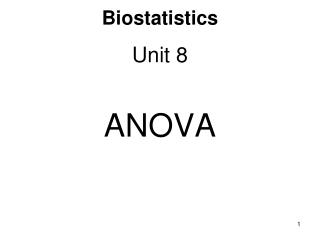Download PresentationBiostatistics

Loading in 2 Seconds...

# Biostatistics - PowerPoint PPT Presentation

Biostatistics. Unit 8 ANOVA. ANOVA—Analysis of Variance. ANOVA is used to determine if there is any significant difference between the means of groups of data. In one-way ANOVA these groups vary under the influence of a single factor . ANOVA—Analysis of Variance.I am the owner, or an agent authorized to act on behalf of the owner, of the copyrighted work described.
Download Presentation## Biostatistics

An Image/Link below is provided (as is) to download presentation

Download Policy: Content on the Website is provided to you AS IS for your information and personal use and may not be sold / licensed / shared on other websites without getting consent from its author.While downloading, if for some reason you are not able to download a presentation, the publisher may have deleted the file from their server.

- - - - - - - - - - - - - - - - - - - - - - - - - - E N D - - - - - - - - - - - - - - - - - - - - - - - - - -
Presentation Transcript
1. Biostatistics Unit 8 ANOVA

2. ANOVA—Analysis of Variance • ANOVA is used to determine if there is any significant difference between the means of groups of data. • In one-way ANOVA these groups vary under the influence of a single factor.

3. ANOVA—Analysis of Variance ANOVA was developed in the 1920s by Ronald A. Fisher (1890-1962) who worked for the British Government Agricultural Department.

4. Data Table • Data for ANOVA are placed in a data table. • There must be at least three groups of data.

5. Assumptions and Hypotheses The assumptions in ANOVA are: -normal distribution of the data -independent simple random samples -constant variance The hypotheses are: H0: all means are equal HA: not all the means are equal

6. Test Statistic • The test statistic is V.R. which is distributed as F with the appropriate number of numerator degrees of freedom and denominator degrees of freedom. • A large value of F indicates rejection of H0.

7. Calculations • Basic calculations are done to determine the values of Sx, Sx2 and n for each group. Place the data from each group in one of the lists of the TI-83. Use of 1-Var Stats gives these values automatically.

8. Calculations 2. An ANOVA table is prepared which includes: df Degrees of freedom SS Sum of squares MS Mean squares F Variance ratio

9. Calculations 3. N and k are used to calculate degrees of freedom. TOTAL df = N – 1 GROUP df = k – 1 ERROR df = N - k

10. Calculations 4. Calculations for ANOVA table values: [A] correction factor [D] SS Error [B] Sum of Squares Total [E] MS Group Value (SS Total) [F] MS Error [C] SS Group [G] F (V.R.)

11. Sample ANOVA Calculations a. Given Opercular breathing rates of goldfish at different temperatures.N = 48 (number of measurements) k = 6 (number of groups)

12. Sample ANOVA Calculations b. Assumptions • normal distribution of data • independent simple random samples • constant variance

13. Sample ANOVA Calculations c. Hypotheses H0: all means are equal HA: not all the means are equal

14. Sample ANOVA Calculations d. Statistical test

15. Sample ANOVA Calculations Decision criteria The critical value of F with 5 numerator degrees of freedom and 42 denominator degrees of freedom is about 2.45 at the 95% confidence level. We reject H0 if V.R. > 2.45.

16. Sample ANOVA Calculations [A] Calculate correction factor Remember: there is a big difference between (Sx)2 and Sx2.

17. Sample ANOVA Calculations [B] Calculate SS Total

18. Sample ANOVA Calculations [C] Calculate SS Group

19. Sample ANOVA Calculations [D] Calculate SS Error

20. Sample ANOVA Calculations [E] Calculate MS Group

21. Sample ANOVA Calculations [F] Calculate MS Error

22. Sample ANOVA Calculations [G] Calculate Variance Ratio Result: the completed ANOVA table

23. Sample ANOVA Calculations f. Discussion • The 95% CI level for F with 5 numerator degrees of freedom and 42 denominator degrees of freedom is 2.45 as read from the F tables. • The actual value is 12.01 with a probability of 2.98 x 10-7. • This means that H0 is rejected.

24. Sample ANOVA Calculations g. Conclusions We conclude that not all the means of the groups are equal.

25. fin